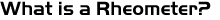A rheometer is an instrument for measuring the flow and shear deformation of materials. The two most widely used laboratory methods of determining rheological data are Capillary Rheometers and Rotational Rheometers. Choosing which piece of equipment to use for a specific application can be simplified by asking the question "Are we interested in material behaviour under simulated processing conditions or discriminating structural differences in compared samples?". A Capillary Rheometer is best suited to the measurement of shear viscosity at process-relevant shear rates, extensional viscosity & pressure dependent viscosity, whereas rotational rheometry is most suited to very low shear rate / viscosity measurement, determining changes in structural properties via low amplitude, controlled strain oscillatory measurements and associated tests such as yield stress analysis & creep / creep recovery.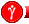Previous of Page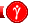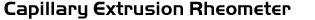A capillary rheometer (Figure 1) is basically a highly instrumented, accurately controlled, ram extruder. The most common configuration is that of a vertically mounted heated barrel containing a capillary die of known, accurately measured, L/D ratio. Above the die entrance situated in the barrel wall is a pressure transducer. A closely fitting piston is driven into the barrel at exactly controlled constant speeds and a pressure drop generated at the capillary die. This pressure drop is allowed to stabilize at which point readings of piston speed and pressure are taken. The speed is then increased to the next pre-determined point and the stabilization sequence repeated. To obtain shear viscosity Vs shear rate curves, a family of speeds / pressure drops are generated (speed dependent on required shear rates and capillary die diameter - Figure 2). Figure 1 Schematic of a Capillary Rheometer in Operation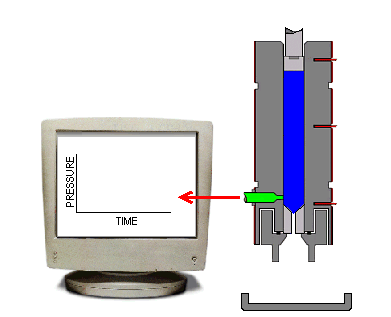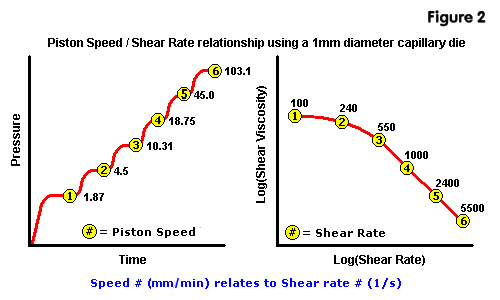Measurements are normally made using two capillary dies - one long die (usually ~20:1 L/D ratio) and a short die (~1:1 L/D ratio). This enables the use of the Bagley end correction formula. The overall pressure drop through a die consists of an entrance pressure, pressure drop along the capillary length (land), and a small exit pressure. The Bagley code removes the entrance & exit pressure effects, the corrected pressure therefore relating to steady state pressure flow within the capillary only. The formula assumes that for the same rate of flow Q, the entrance and exit pressure drops for two dies of the same diameter but different lengths are the same. Instead of calculating shear stress, t, using the formula: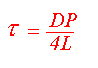Where D is the capillary diameter, L the length and P pressure, the difference in pressures between the long and short dies is employed: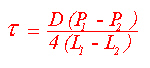For the processing calculations used in injection moulding flow simulation packages, corrected data is essential since flow channels can be long, continuous, and thin. Uncorrected viscosities measured on a single capillary die would over estimate the pressure drop and therefore the viscosity.
 The rheometer can cover a wide shear rate range, for example, with a 1mm diameter capillary die a range of ~1 to 50,000 1/s can be achieved. Higher or lower ranges can be achieved by altering the die diameter - doubling the diameter reduces the shear rate range by a factor of 8, conversely halving the diameter increases the range by a factor of 8. The shear stress is also related to the die geometry - doubling the capillary length halves the shear stress and so on. The capillary rheometer is also used for other applications such as the determination of Slippage, Extensional Viscosity & hydrostatic pressure effects on viscosity.Previous of Page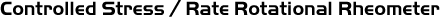A controlled stress rotational rheometer is an extremely sensitive, yet robust bench top instrument which is available in a number of machine configurations. The business end of the rheometer consists of a range of modular measurement systems comprising of either cone & plate, plate / plate or coaxial cylinder configurations (Figures 3a, 3b & 3c). Measurement geometries are connected to a spindle which is driven via a non contact induction motor. The drive system is supported by a high quality porous carbon air bearing which results in virtually frictionless movement of the measurement system. Angular deflection is detected via a low inertia optical encoder to high accuracy. Temperature of the sample is controlled by an environment controlled heated clamshell oven & baseplate.
 Figure 3a - Cone & Plate Figure 3b - Plate / Plate Figure 3c - Coaxial Cylinder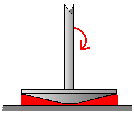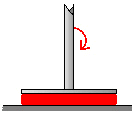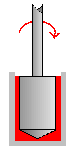Measurement Techniques Rotational Measurements In continuous rotation testing the machine can be operated in two main measurement modes: Controlled Stress & Controlled Rate. In Controlled Stress measurements a known torque (stress) is applied to the sample and the resultant displacement (strain or strain rate) is measured, (Figure 2). In controlled rate mode a defined speed (shear rate) is applied and the resultant torque (stress) is measured. Constant rotation is the mode used to derived shear viscosity Vs shear rate data. Oscillatory Measurements Unlike constant rotation (shear) measurements, oscillation is a non-destructive technique which is ideal for investigating structure / structural changes in materials. The oscillation technique involves applying a sinusoidal oscillating stress (t)wave to a material and measuring the resulting strain wave (g). A purely elastic (Hookean solid) material will retain all of the deformation energy applied to it and will therefore have a phase difference (phase angle) of 0 degrees, (Figure 4a). Conversely, a purely viscous (Newtonian) fluid dissapates all of the applied energy and will have a phase angle of 90 degrees, (Figure 4b). Polymeric materials are Visco-elastic and as such their responses fall somewhere between these two extremes (Figure 4c).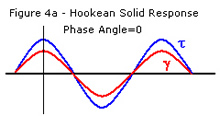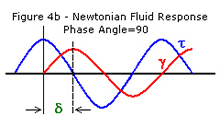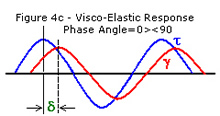Measurements are made over a range of frequencies and a family of curves generated. Data of most interest derived from these oscillation strain control measurements are Complex Viscosity (h*), Phase angle (°), and Storage and Loss modulus (G' & G"), as a function of frequency, (Figure 5).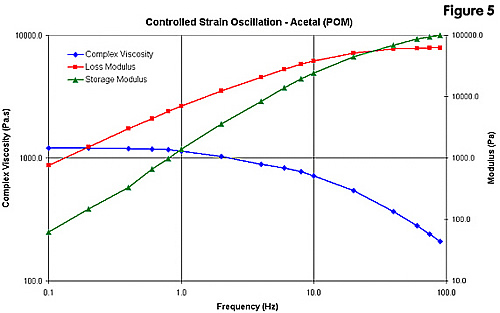The limiting (plateau) viscosity reached at low frequency is indicative of a material's zero shear viscosity and therefore it's molecular weight. The point at which the G' & G" curves cross over can also be a good indication of magnitude of molecular weight and molecular weight distribution, (Figures 6a & 6b). The relationship of G' to G" describes the visco-elastic response of the material. Phase angle or Tan phase (Tan Delta) is a good indicator of phase transitions (e.g. Tg) when measurements are made as a function of temperature.
 Figure 6a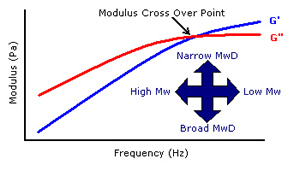Figure 6b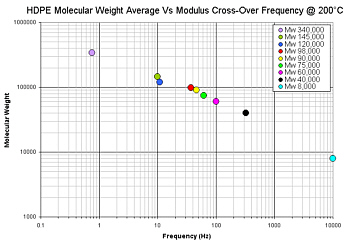For these reasons the measurement is ideal for detecting discrete changes in sample structure and can be used for discriminating batch to batch variance in pre-process QC testing of bought-in materials, degradation of materials during processing resulting in chain scission / cross linking and failures in service due to e.g. UV / thermal / chemical degradation. Materials which cannot be dissolved to enable molecular characterisation by Gas Permeability Chromatography (GPC) such as Acetal (POM) & ECTFE can also be easily characterised using oscillatory rheometry.